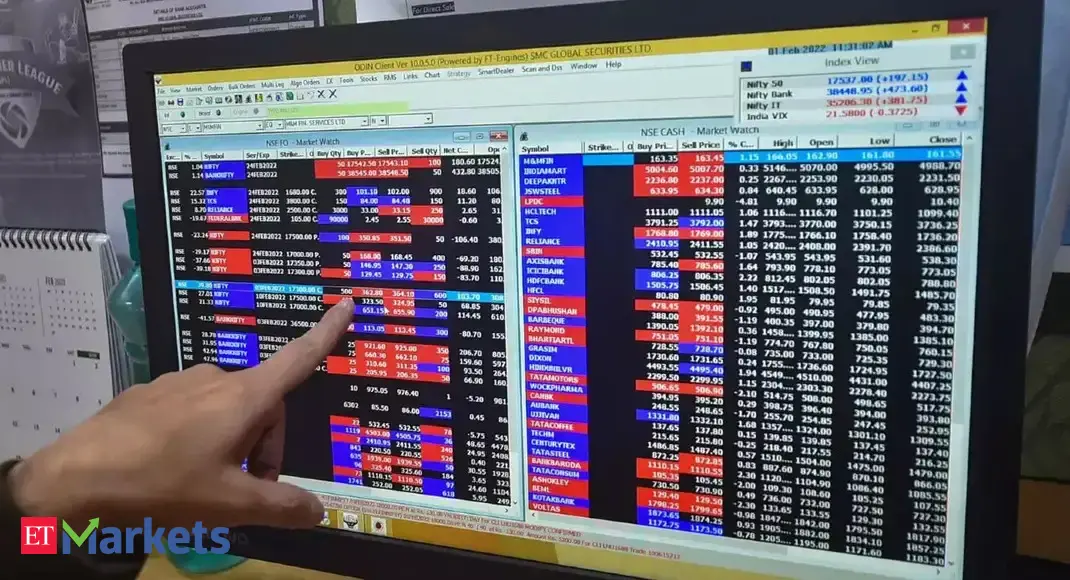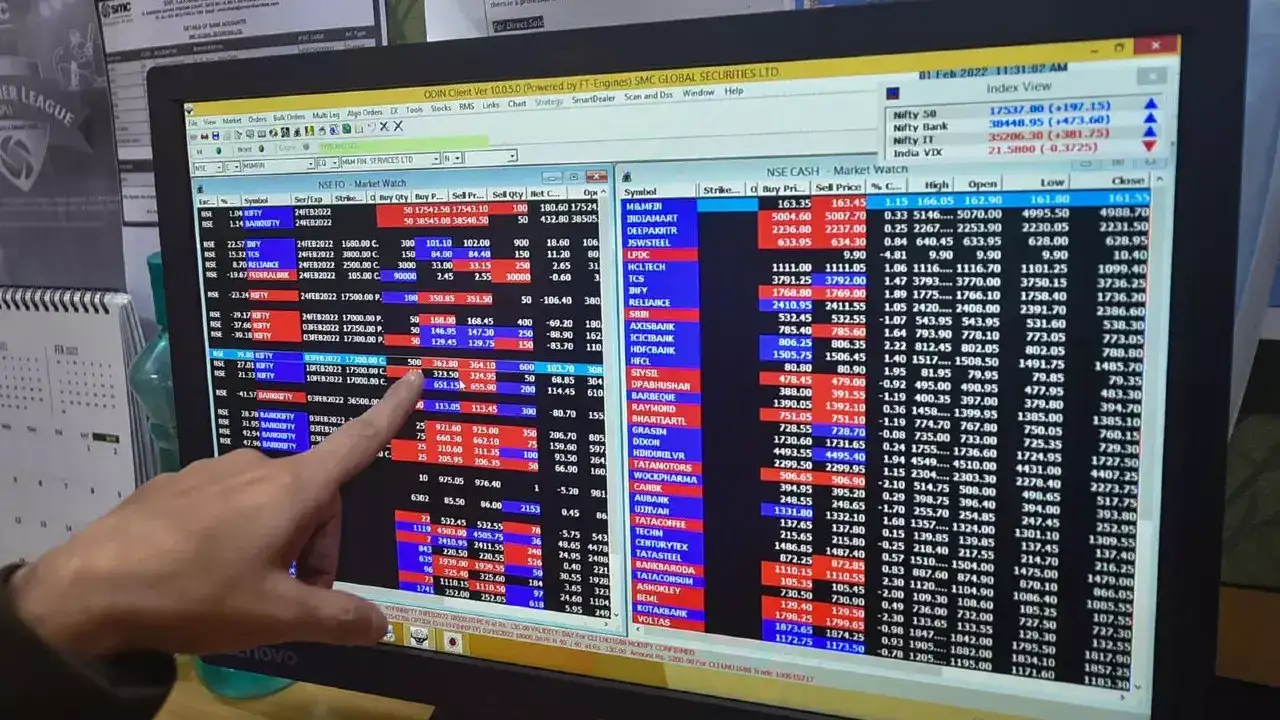# How to book timely profits using disparity ratio

## The methodology that we would discuss is not a random one; it is based upon statistics and hence, it would help you take decisions wisely.So, if you are eager to know about that crystal ball that will help you to book timely profits then, let me tell you, that’s the disparity index (or disparity ratio).The methodology that we would discuss is not a random one; it is based upon statistics and hence, it would help you take decisions wisely.So, if you are eager to know about that crystal ball that will help you to book timely profits then, let me tell you, that’s the disparity index (or disparity ratio).